Online Tution   »   Sample Papers   »   CBSE Class 10th Maths Sample Paper...

# CBSE Class 10th Maths Term 2 Sample Paper with Solutions

## Class 10 Maths Term 2 Sample Paper

Central Board of Secondary Education has published the CBSE Class 10 Maths Sample Paper 2021-22 on its official website on 19th January 2022 on the official website https://www.cbse.gov.in/. The Central Board of Secondary Education will conduct CBSE Term 2 examination for Class 10th and 12th in March-April 2022. The candidates who are going to appear in CBSE Term 2 examination must solve the CBSE Class 10 Maths Sample Paper 2021-22 given on this page. Through CBSE Class 10 Maths Sample Paper 2021-22, the candidates will get to know about the difficulty level of the CBSE Term 2 examination. In this article, we have given CBSE Class 10 Maths Term-2 Sample Paper 2022. The candidates must solve the CBSE Class 10 Maths Sample Paper 2021-22 given on this page and bookmark this page to get all the updates.

Check Out: CBSE Class 10 Maths Term 2 Important Questions

Check Out: CBSE Class 10 Maths Term 2 Previous Year Papers

Check Out: CBSE Class 10 English Term 2 Exam Analysis 2022

Check Out: CBSE Class 10 SST Previous Year Papers

Check Out: CBSE Term 2 Exam Sheet 2022

## CBSE Class 10th Maths Term 2 Most Important Questions

Read: Tips to get 95+ in Term 2 Class 10 Maths## CBSE Class 10th Maths Sample Paper 2022 Term-2: Exam Pattern

Here we have discussed the CBSE Class 10 Maths Term-2 exam pattern. The candidates appearing in the CBSE Term 2 examination must check the exam pattern given below:

Exam Pattern for Basic Maths

1. The Basic Maths exam of Class 10th Term 2 consists of 14 questions.
2. The Basic Maths is divided into three sections namely A, B, C.
3.  Section A: In Section A, 6 questions will be asked. Each question carries 2 marks.
4. Section B: In Section B, 4 questions will be asked. Each question carries 3 marks.
5. Section C: In Section C, 4 questions will be asked. Each question carries 4 marks.

Exam Pattern for Standard Maths

1. The Standard Maths exam of Class 10th Term 2 consists of 14 questions.
2. The Standard Maths is divided into three sections namely A, B, C.
3.  Section A: In Section A, 6 questions will be asked. Each question carries 2 marks.
4. Section B: In Section B, 4 questions will be asked. Each question carries 3 marks.
5. Section C: In Section C, 4 questions will be asked. Each question carries 4 marks.

Are you in class12th/passed and willing to get admission in TOP COLLEGE of India ?

Download the CBSE Maths Sample Paper 2022 Class 10 by following the below-mentioned steps

Step II- Click on the notification appearing in the academic section- “Sample Question Papers of Classes X for Term 2 Exams 2022”.

Step III- Now click on the link mentioned under “Sample Papers Class X”.

Step IV- The list of all subjects “Class X Sample Question Paper & Marking Scheme for Exam 2022” appears on the screen.

Step V- Click on “SQP” for “Maths” and download CBSE Class 10th Maths Sample Paper Term 2 pdf along with Answer Key.

Step VI- Check the marking scheme after attempting each subject CBSE Class 10th Maths Sample Paper 2022 Term 2.

## Class 10 Maths Term 2 Sample Papers: Important Questions of Basic Math

Below we have given the CBSE Class 10 Maths Term-2 Sample Paper 2022. Solve the CBSE Class 10th Maths Term-2  Sample Paper 2022 and assess your preparation:

1. Find the roots of the quadratic equation 3𝑥 2 − 7𝑥 − 6 = 0.

OR

Find the values of k for which the quadratic equation 3𝑥 2 + 𝑘𝑥 + 3 = 0 has real and equal roots.

Solution:

3𝑥 2 − 7𝑥 − 6 = 0

⇒ 3𝑥 2 − 9𝑥 + 2𝑥 − 6 = 0

⇒ 3𝑥(𝑥 − 3) + 2(𝑥 − 3) = 0

⇒ (𝑥 − 3)(3𝑥 + 2) = 0

∵ 𝑥 = 3, − 2/3

OR

Since the roots are real and equal,

∴ 𝐷 = 𝑏 2 − 4𝑎𝑐 = 0

⇒k 2 – 4×3×3 = 0 (∵ 𝑎 = 3, 𝑏 = 𝑘, 𝑐 = 3)

⇒k 2 = 36

⇒k = 6 𝑜𝑟 −6

2.  Three cubes each of volume 64cm3 are joined end to end to form a cuboid. Find the total surface area of the cuboid so formed?

Solution:

Let 𝑙 be the side of the cube and L, B, H be the dimensions of the cuboid Since 𝑙 3 = 64 𝑐𝑚3 ∴ 𝑙 = 4 𝑐𝑚

Total surface area of cuboid is 2[𝐿𝐵 + 𝐵𝐻 + 𝐻𝐿], Where L=12, B=4 and H=4

=2(12 × 4 + 4 × 4 + 4 × 12) 𝑐𝑚2 = 224𝑐𝑚2

3.  An inter-house cricket match was organized by a school. The distribution of runs made by the students is given below. Find the median runs scored.

 Runs scored 0-20 20-40 40-60 60-80 80-100 Number of students 4 6 5 3 4

Solution:

 Runs scored Frequency Cumulative Frequency 0-20 4 4 20-40 6 10 40-60 5 15 60-80 3 18 80-100 4 22

Total frequency (N) = 22

𝑁/2 = 11; So 40-60 is the median class.

Median = 𝑙 + ( 𝑁 2 )−𝑐𝑓 𝑓 × ℎ

= 40 + 11−10/ 5 x 20

= 44 runs

4.  Find the common difference of the AP 4,9,14,… If the first term changes to 6 and the common difference remains the same then write the new AP.

Solution

The common difference is 9 – 4=5

If the first term is 6 and the common difference is 5, then the new AP is,

6, 6+5, 6+10…

=6,11,16….

5.  The mode of the following frequency distribution is 38. Find the value of x

 Runs scored 0-10 10-20 20-30 30-40 40-50 60-70 Number of students 7 9 12 16 x 11

Solution:

∵ Mode = 38.

∴ The modal class is 30-40.

Mode = 𝑙 + 𝑓1− 𝑓0 /2𝑓1−𝑓0−𝑓2 × ℎ

=30 + 16−12/ (32−12−𝑥 )x10 = 38

4/ 20−𝑥 x 10 =8

8(20-x) = 40

20-x= 5

X= 15

Q.6 XY and MN are the tangents drawn at the endpoints of the diameter DE of the circle with center O. Prove that XY  MN.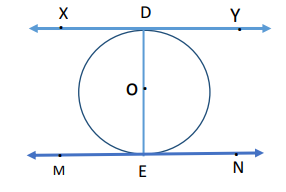OR

In the given figure, a circle is inscribed in the quadrilateral ABCD. Given AB=6cm, BC=7cm and CD=4cm. Find AD.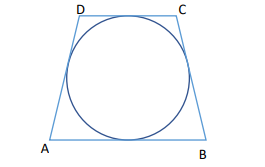Solution:∵XY is the tangent to the circle at the point D

∴ OD  XY   ODX = 900

EDX = 900

Also, MN is the tangent to the circle at E

∴ OE  MN  OEN = 900   DEN = 900

⇒  EDX =  DEN (𝑒𝑎𝑐ℎ 900 ).

which are alternate interior angles.

∴ XY || MN

OR∵Tangent segments drawn from an external point to a circle are equal

∴ BP=BQ

CR=CQ

DR=DS

AP=AS

⇒BP+CR+DR+AP = BQ+CQ+DS+AS

= 3 cm

7. An AP 5, 8, 11…has 40 terms. Find the last term. Also find the sum of the last 10 terms.

Solution:

First Term of the AP(a) = 5

Common difference (d) = 8-5=3

Last term = 𝑎40 = a+(40-1) d = 5 + 39 × 3 = 122

Also 𝑎31 = 𝑎 + 30𝑑 = 5 + 30 × 3 = 95

Sum of last 10 terms = 𝑛 2 (𝑎31 + 𝑎40) = 10

2 (95 + 122) = 5 × 217 = 1085

8. A tree is broken due to the storm in such a way that the top of the tree touches the ground and makes an angle of 300 with the ground. The length of the broken upper part of the tree is 8 meters. Find the height of the tree before it was broken.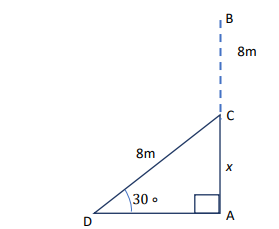OR

Two poles of equal height are standing opposite each other on either side of the road 80m wide. From a point between them on the road the angles of elevation of the top of the two poles are respectively 600 and 300 . Find the distance of the point from the two poles.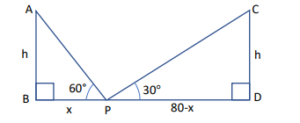Solution:Let, AB be the tree broken at C,

Also let 𝐴𝐶 = 𝑥

In ∆ CAD, sin300 = 𝐴𝐶

𝐷𝐶 ⇒ 1 2 = 𝑥 8

⇒ 𝑥 = 4 𝑚

⇒the length of the tree is = 8+4 =12m

ORLet AB and CD be two poles of height h meters also let P be a point between them on the road which is x meters away from foot of first pole AB, PD= (80-x) meters.

In ∆ABP, 𝑡𝑎𝑛60𝑜 = ℎ 𝑥

⇒ ℎ = 𝑥√3 .…(1)

In ∆CDP, 𝑡𝑎𝑛 30𝑜 = ℎ 80−𝑥

⇒ ℎ = 80−𝑥 √3 ….(2) 𝑥√3 = 80 − 𝑥 √3 [∵ 𝐿𝐻𝑆(1) = 𝐿𝐻𝑆(2), 𝑠𝑜 𝑒𝑞𝑢𝑎𝑡𝑖𝑛𝑔 𝑅𝐻𝑆] ⇒ 3𝑥 = 80 − 𝑥

⇒ 4𝑥 = 80

⇒ 𝑥 = 20𝑚 So, 80 − 𝑥 = 80 − 20 = 60𝑚

Hence the point is 20m from one pole and 60 meters from the other pole.

9. PA and PB are the tangents drawn to a circle with center O. If PA= 6 cm and  APB=600, then find the length of the chord AB.

Solution:

PA = PB (Tangent segments drawn to a circle from an external point are equal)

∴ In ∆𝐴𝑃𝐵,  PAB =  PBA

Also,  APB = 600

In ∆𝐴𝑃𝐵, sum of three angles is 1800 .

Therefore,  PAB +  PBA = 1800 –  APB= 1800 – 600 = 1200 .

∴ PAB =  PBA = 600 (∵ PAB =  PBA)

∵ ∆𝐴𝑃𝐵 is an equilateral triangle.

So, 𝐴𝐵 = 6𝑐𝑚

10.  The sum of the squares of three positive numbers that are consecutive multiples of 5 is 725. Find the three numbers.

Solution:

Let the three consecutive multiples of 5 be 5x, 5x+5, 5x+10.

Their squares are (5𝑥) 2 , (5𝑥 + 5) 2and(5𝑥 + 10) 2 .

(5𝑥) 2 + (5𝑥 + 5) 2 + (5𝑥 + 10) 2 = 725

⇒25𝑥 2 + 25𝑥 2+ 50x + 25 + 25𝑥 2 + 100x + 100 = 725

⇒ 75𝑥 2 + 150𝑥 − 600 = 0

⇒ 𝑥 2 + 2𝑥 − 8 = 0

⇒ (𝑥 + 4)(𝑥 − 2) = 0

⇒ 𝑥 = −4, 2

⇒ 𝑥 = 2 (ignoring –ve value)

So the numbers are 10, 15 and 20

11. Construct two concentric circles of radii 3cm and 7cm. Draw two tangents to the smaller circle from a point P which lies on the bigger circle.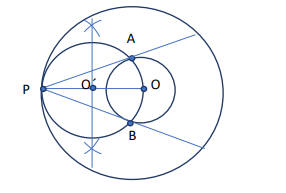OR

Draw a pair of tangents to a circle of radius 6cm which are inclined to each other at an angle of 600 . Also find the length of the tangent.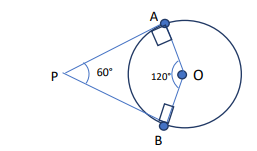Solution:

Draw two concentric circles with center O and radii 3cm and 7cm respectively. Join OP and bisect it at 𝑂 ′ , so 𝑃𝑂′ = 𝑂 ′𝑂

Construct circle with center 𝑂 ′ and radius 𝑂 ′𝑂

Join PA and PBOR

Draw a circle of radius 6cm

Draw OA and Construct ∠ 𝐴𝑂𝐵 = 1200

Draw ∠ 𝑂𝐴𝑃 = ∠ 𝑂𝐵𝑃 = 900

PA and PB are required tangents

Join OP and apply tan∠𝐴𝑃𝑂 = tan 30° = 6 𝑃𝐴

⇒ Length of tangent = 6√3 cm12.  The following age-wise chart of 300 passengers flying from Delhi to Pune is prepared by the Airlines staff. Find the mean age of the passengers.

 Age Less than 10 Less than 20 Less than 30 Less than 40 Less than 50 Less than 60 Less than 70 Less than 80 Number of passengers 14 44 82 134 184 245 287 300

Solution: Converting the cumulative frequency table into exclusive classes, we get:

 Age No of passengers(fi) xi fi xi 0-10 14 5 70 10-20 30 15 450 20-30 38 25 950 30-40 52 35 1820 40-50 50 45 2250 50-60 61 55 3355 60-70 42 65 2730 70-80 13 75 975 ∑ 𝑓𝑖 = 300 ∑ 𝑓𝑖𝑥𝑖 =12600

Mean age = 𝑥̅= ∑ 𝑓𝑖𝑥𝑖 ∑ 𝑓𝑖 = 12600 300

𝑥̅= 42

13. A lighthouse is a tall tower with light near the top. These are often built on islands, coasts or on cliffs. Lighthouses on water surface act as a navigational aid to the mariners and send warning to boats and ships for dangers. Initially wood, coal would be used as illuminators. Gradually it was replaced by candles, lanterns, electric lights. Nowadays they are run by machines and remote monitoring. Prongs Reef lighthouse of Mumbai was constructed in 1874-75. It is approximately 40 meters high and its beam can be seen at a distance of 30 kilometres. A ship and a boat are coming towards the lighthouse from opposite directions. Angles of depression of flash light from the lighthouse to the boat and the ship are 30 0 and 60 0 respectively.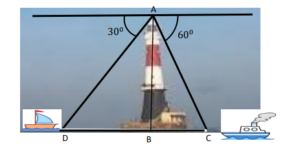i) Which of the two, boat or the ship is nearer to the light house. Find its distance from the lighthouse?

ii) Find the time taken by the boat to reach the light house if it is moving at the rate of 20 km per hour.

Solution:

I)The ship is nearer to the lighthouse as its angle of depression is greater.

In ∆ ACB, tan 600 = 𝐴𝐵 /𝐵𝐶

√3 = 40 𝐵𝐶

∴ BC = 40 √3

= 40√3 3 m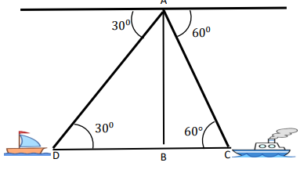II)In ∆ ADB, tan 30 0 = 𝐴𝐵 𝐵𝐷

⇒ 1 √3 = 40 𝐷𝐵

∴ DB = 40√3𝑚

Time taken to cover this distance = ( 60 2000 × 40√3) minutes

= 60√3 100 = 2.076 minutes

Q.14 Krishnanagar is a small town in Nadia District of West Bengal. Krishnanagar clay dolls are unique in their realism and quality of their finish. They are created by modelling coils of clay over a metal frame. The figures are painted in natural colours and their hair is made either by sheep’s wool or jute. Artisans make models starting from fruits, animals, God, goddess, farmer, fisherman, weavers to Donald Duck and present comic characters. These creations are displayed in different national and international museums. Here are a few images (not to scale) of some clay dolls of Krishnanagar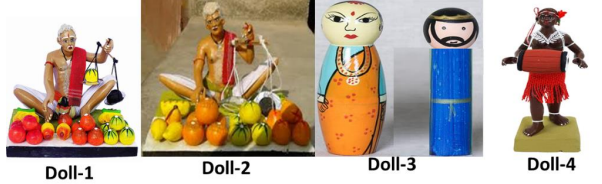The ratio of diameters of red spherical apples in Doll-1 to that of spherical oranges in Doll-2 is 2:3. In Doll-3, male doll of blue colour has cylindrical body and a spherical head. The spherical head touches the cylindrical body. The radius of both the spherical head and the cylindrical body is 3cm and the height of the cylindrical body is 8cm. Based on the above information answer the following questions:

i) What is the ratio of the surface areas of red spherical apples in Doll-1 to that of spherical oranges in Doll-2.?

ii) The blue doll of Doll-3 is melted and its clay is used to make the cylindrical drum of Doll-4. If the radius of the drum is also 3cm, find the height of the drum.

Solution:

I) Let 𝑟1𝑎𝑛𝑑 𝑟2 be respectively the radii of apples and oranges

∵ 2𝑟1: 2𝑟2 = 2: 3 ⇒ 𝑟1: 𝑟2 = 2: 3

4𝜋𝑟1 2 : 4𝜋𝑟2 2 = ( 𝑟1 𝑟2 ) 2 = ( 2 3 ) 2 = 4: 9

II)Let the height of the drum be h. Volume of the drum = volume of the cylinder + volume of the sphere π3 2h = (π3 2 × 8 + 4 3 π3 3 ) 𝑐𝑚3

⇒ ℎ = (8 + 4)𝑐𝑚

⇒ ℎ = 12𝑐𝑚

## Sample Paper Class 10 Maths Term 2: Important Questions of Standard Maths

Q. 1 Find the value of a25 – a15 for the AP: 6, 9, 12, 15, ………..

OR

If 7 times the seventh term of the AP is equal to 5 times the fifth term, then
find the value of its 12th term.

Solution:

a = 6, d = 3 ; a25 = 6 + 24(3) = 78
a15 = 6 + 14(3) = 48 ; a25 – a15 = 78 – 48 = 30
OR
7(𝑎 + 6𝑑) = 5(𝑎 + 4𝑑)
⇒2𝑎 + 22𝑑 = 0

⇒ 𝑎 + 11𝑑 = 0 ⇒ 𝑡12 = 0

Q.2 If Ritu were younger by 5 years than what she really is, then the square of her
age would have been 11 more than five times her present age. What is her
present age?
OR
Solve for x: 9x² – 6px + (p² – q²) = 0

Solution:

Let the present age of Ritu be 𝑥 years

(𝑥 − 5)^2 = 5𝑥 + 11

𝑥 2 − 15𝑥 + 14 = 0½

(𝑥 − 14)(𝑥 − 1) = 0 ⇒ 𝑥 = 1 𝑜𝑟 14

x = 14 years (rejecting 𝑥 = 1 as in that case Ritu’s age 5 years ago will be –ve )

OR

9𝑥 2 − 6𝑝𝑥 + (𝑝 2 − 𝑞 2) = 0

𝑎 = 9, 𝑏 = −6𝑝, 𝑐 = 𝑝 2 − 𝑞 2

𝐷 = 𝑏 2 − 4𝑎𝑐 = (−6𝑝) 2 − 4(9)(𝑝 2 − 𝑞 2) = 36𝑞 2

𝑥 = −𝑏 ± √𝐷/2𝑎 = 6𝑝 ± 6𝑞/18 = 𝑝 + 𝑞/3 𝑜𝑟 𝑝 − 𝑞/3

Q. 3 The curved surface area of a right circular cone is 12320 cm². If the radius of its
base is 56cm, then find its height

CSA (cone) = 𝜋𝑟𝑙 = 12320
22/7× 56 × 𝑙 = 12320
𝑙 = 70 𝑐𝑚
ℎ = √702 − 562 = 42 cm

Q. 4 Find the value of 𝑚 so that the quadratic equation 𝑚𝑥(5𝑥 − 6) = 0 has two
equal roots.

5mx² – 6mx + 9 = 0
b² – 4ac = 0 ⇒ (-6m)² – 4(5m)(9) = 0
⇒ 36m(m – 5) = 0
⇒ m = 0, 5 ; rejecting m=0, we get m = 5

Q. 5 From a point P, two tangents PA and PB are drawn to a circle C(0, r).
If OP = 2r, then find ∠𝐴𝑃𝐵. What type of triangle is APB?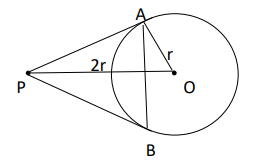Solution:let ∠𝐴𝑃𝑂 = 𝜃 𝑆𝑖𝑛𝜃 = 𝑂𝐴 𝑂𝑃 = 1 2

⇒ 𝜃 = 300

⇒ ∠𝐴𝑃𝐵 = 2𝜃 = 600

Also ∠𝑃𝐴𝐵 = ∠𝑃𝐵𝐴 = 600 (∵ 𝑃𝐴 = 𝑃𝐵)

⇒△ 𝐴𝑃𝐵 is equilateral

Q.6 Mrs. Garg recorded the marks obtained by her students in the following table.
She calculated the modal marks of the students of the class as 45. While
printing the data, a blank was left. Find the missing frequency in the table given
below

 Marks Obtained 0 – 20 20 – 40 40-60 60-80 80-100 Number of Students 5 10 — 6 3

Solution:

Modal class is 40 − 60, 𝑙 = 40, ℎ = 20, 𝑓1 =? , 𝑓0 = 10, 𝑓2 = 6

45 = 40 + 20 × [ 𝑓1 − 10 2𝑓1 − 10 − 6 ]

⇒ 1 4 = 𝑓 1 −10 2𝑓 1 −16

⇒2𝑓1 − 16 = 4𝑓1 − 40

⇒ 𝑓1 = 12

Q. 7 Following is the distribution of the long jump competition in which 250 students
participated. Find the median distance jumped by the students. Interpret the
median

 Distance (in m) 0 – 1 1 – 2 2 – 3 3 – 4 4 – 5 Number of Students 40 80 62 38 30

Solution:

 Distance (in m) 0 – 1 1 – 2 2 – 3 3 – 4 4 – 5 Number of Students 40 80 62 38 30 𝑐f 40 120 182 220 250

𝑛 2 = 250 2 = 125

⇒ 𝑚𝑒𝑑𝑖𝑎𝑛 𝑐𝑙𝑎𝑠𝑠 𝑖𝑠 2 − 3, 𝑙 = 2, ℎ = 1, 𝑐𝑓 = 120, 𝑓 = 62

𝑚𝑒𝑑𝑖𝑎𝑛 = 𝑙 + 𝑛 2 − 𝑐𝑓/𝑓 × 𝑖

= 2 + 5/62

= 129/62 = 2 5 62 𝑚 𝑜𝑟 2.08𝑚

50% of students jumped below2 5 62 m and 50% above it.

Q.8 Construct a pair of tangents to a circle of radius 4cm, which are inclined to
each other at an angle of 60°.

Solution:

Draw a circle of radius 4cm
Draw OA and construct ∠𝐴𝑂𝐵 = 1200
Draw ∠𝑂𝐴𝑃 = ∠𝑂𝐵𝑃 = 900
PA and PB are required tangents

Q.9 The distribution given below shows the runs scored by batsmen in one-day
cricket matches. Find the mean number of runs.

 Runs scored 0 – 40 40 – 80 80 – 120 120 – 160 160 – 200 Number of batsmen 12 20 35 30 23

Solution:

 Runs scored 0 – 40 40 – 80 80 – 120 120 – 160 160 – 200 Total Number of batsmen 12 20 35 30 23 120 Xi 20 60 100 140 180 fi xi 13280

𝑚𝑒𝑎𝑛 ( 𝑥̅) = ∑ 𝑓𝑖 𝑥𝑖 /∑ 𝑓𝑖 = 13280/120 = 110.67 𝑟𝑢𝑛s

Q. 10 Two vertical poles of different heights are standing 20m away from each other
on the level ground. The angle of elevation of the top of the first pole from the
foot of the second pole is 60° and angle of elevation of the top of the second
pole from the foot of the first pole is 30°. Find the difference between the heights
of two poles. (Take √3 = 1.73)
OR
A boy 1.7 m tall is standing on a horizontal ground, 50 m away from a building.
The angle of elevation of the top of the building from his eye is 60°. Calculate
the height of the building. (Take √3 = 1.73)

Solution: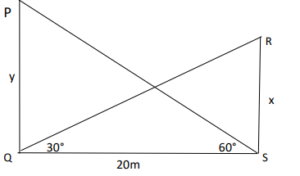𝐼𝑛 ∆𝑃𝑄𝑆, 𝑡𝑎𝑛600 = 𝑦 20 ⇒ 𝑦 = 20√3m

𝐼𝑛 ∆𝑅𝑆𝑄, 𝑡𝑎𝑛300 = 𝑥 20 ⇒ 𝑥 = 20 √3 𝑚

𝑦 − 𝑥 = 20√3 − 20 √3 = 40 √3 = 40√3 3 = 23.06m

OR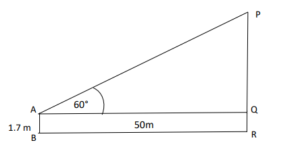Let PR be the building and AB be the boy

𝐼𝑛 ∆𝑃𝑄𝑅,𝑡𝑎𝑛600 = 𝑃𝑄 50 ⇒ 𝑃𝑄 = 50√3𝑚

Height of the building = 𝑃𝑅 = (50√3 + 1.7)𝑚 = 88.2m

Q.11 The internal and external radii of a spherical shell are 3cm and 5cm
respectively. It is melted and recast into a solid cylinder of diameter 14cm, find
the height of the cylinder. Also find the total surface area of the cylinder. (Take 𝜋 =22/7)

Solution:

Volume of shell = Volume of cylinder

⇒ 4𝜋 3 [ 5³ − 3³] = 𝜋(7) 2ℎ

⇒ ℎ = 8 3 = 2 2 3 𝑐m

TSA of cylinder is = 2𝜋𝑟(𝑟 + ℎ) = 2 × 22 7 × 7 × (7 + 8 3 ) = 44 × 29 3 = 1276 3 𝑐𝑚2 𝑜𝑟 425.33𝑐𝑚2

Q.12 Prove that the angle between the two tangents drawn from an external point to
a circle is supplementary to the angle subtended by the line segment joining the
points of contact to the centre.
OR
Two tangents TP and TQ are drawn to a circle with centre O from an external
point T. Prove that ∠𝑃𝑇𝑄 = 2∠𝑂𝑃Q

Solution: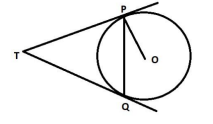∠𝑂𝐴𝑃 + ∠𝑂𝐵𝑃 + ∠𝐴𝑃𝐵 + ∠𝐴𝑂𝐵 = 360𝑜
⇒ 900 + 900 + ∠𝐴𝑃𝐵 + ∠𝐴𝑂𝐵 = 360𝑜 (∵ 𝑇𝑎𝑛𝑔𝑒𝑛𝑡 ⊥ 𝑟𝑎𝑑𝑖𝑢𝑠)
⇒ ∠𝐴𝑃𝐵 + ∠𝐴𝑂𝐵 = 1800

OR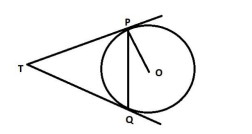Let ∠𝑃𝑇𝑄 = 𝜃 𝑇𝑃𝑄 is an isosceles triangle.

∠𝑇𝑃𝑄 = ∠𝑇𝑄𝑃 = 1 2 (180𝑜 − 𝜃) = 90° − 𝜃/2

∠𝑂𝑃𝑇 = 90𝑜

∠𝑂𝑃𝑄 = ∠𝑂𝑃𝑇 − ∠𝑇𝑃𝑄 = 90𝑜 − (90𝑜 − 𝜃/2 ) = 𝜃/2

∠𝑂𝑃𝑄 = 1/2 ∠𝑃𝑇𝑄

2∠𝑂𝑃𝑄 = ∠𝑃𝑇Q

Q.13 Trigonometry in the form of triangulation forms the basis of navigation, whether
it is by land, sea or air. GPS a radio navigation system helps to locate our
position on earth with the help of satellites.
A guard, stationed at the top of a 240m tower, observed an unidentified boat
coming towards it. A clinometer or inclinometer is an instrument used for
measuring angles or slopes(tilt). The guard used the clinometer to measure the
angle of depression of the boat coming towards the lighthouse and found it to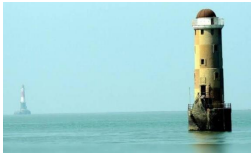(Lighthouse of Mumbai Harbour. Picture credits – Times of India Travel)
i) Make a labelled figure on the basis of the given information and calculate
the distance of the boat from the foot of the observation tower.
ii) After 10 minutes, the guard observed that the boat was approaching the
tower and its distance from tower is reduced by 240(√3 – 1) m. He
immediately raised the alarm. What was the new angle of depression of
the boat from the top of the observation tower?

Solution: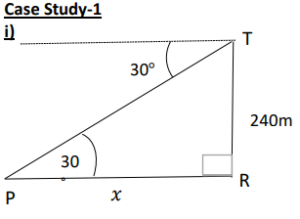In ∆𝑃𝑇𝑅, tan 30𝑜 = 240 𝑥 ⇒ 𝑥 = 240√3 m

ii) Distance of boat from tower= 240√3 − 240(√3 − 1) = 240𝑚

Let the angle of depression = 𝜃

𝑡𝑎𝑛𝜃 = 240 240 = 1 ⇒ 𝜃 = 45𝑜

Q.14 Case Study-2
Push-ups are a fast and effective exercise for building strength. These are
helpful in almost all sports including athletics. While the push-up primarily
targets the muscles of the chest, arms, and shoulders, support required from
other muscles helps in toning up the whole body.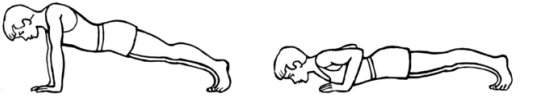Nitesh wants to participate in the push-up challenge. He can currently make
3000 push-ups in one hour. But he wants to achieve a target of 3900 push-ups
in 1 hour for which he practices regularly. With each day of practice, he is able
to make 5 more push-ups in one hour as compared to the previous day. If on
first day of practice he makes 3000 push-ups and continues to practice regularly
till his target is achieved. Keeping the above situation in mind answer the
following questions:
i) Form an A.P representing the number of push-ups per day and hence
find the minimum number of days he needs to practice before the day
his goal is accomplished?
ii) Find the total number of push-ups performed by Nitesh up to the day his
goal is achieved.

Solution:

1. i) 3000, 3005, 3010, …,3900.

𝑎𝑛 = 𝑎 + (𝑛 − 1)𝑑

3900 = 3000 + (n – 1)5

⇒ 900 = 5𝑛 − 5

⇒ 5𝑛 = 905

⇒ 𝑛 = 181 Minimum number of days of practice = 𝑛 − 1 = 180 𝑑𝑎𝑦𝑠

1. ii) 𝑆𝑛 = 𝑛 2 (𝑎 + 𝑙) = 181 2 × (3000 + 3900)

= 624450 pushups

## Class 10 Maths Term 2 Sample Paper Video Solution

CBSE Class 10 Maths Deleted Syllabus 2021-22 for Term 1 & 2

CBSE Class 10 Science Deleted Syllabus 2021-22 for Term 1 & 2

CBSE Term 1 Result 2021-2022 Check @ cbse.gov.in

CBSE Class 12 Physics Deleted Syllabus 2021-22 for Term 1 & 2

When will CBSE Term 1 Result 2022 will be declared?

Related Post:

## CBSE Term 2 Class 10th Maths Sample Paper 2022: FAQs

Q. Where can I get CBSE Class 10th Maths Sample Paper 2022?

On this page, you will get CBSE Class 10th Maths Sample Paper 2022.

Q. When will CBSE announce the CBSE Term 1 Result 2021-22?

The CBSE is going to announce CBSE Term 1 Result 2021-22 soon. The CBSE Term 1 Result 2021-22 can be announced at any time in the 2nd or 3rd week of February 2022.

Q. What are the passing criteria of the CBSE Term 1 exam?

To pass the CBSE Term 1 Examination, students must have scored 33% of the total marks in each subject.

Sharing is caring!

Thank You, Your details have been submitted we will get back to you.